Return to Free Library

Return to Number Menu

We will now discuss the preeminent symbol of the Hexad – the Hexagon.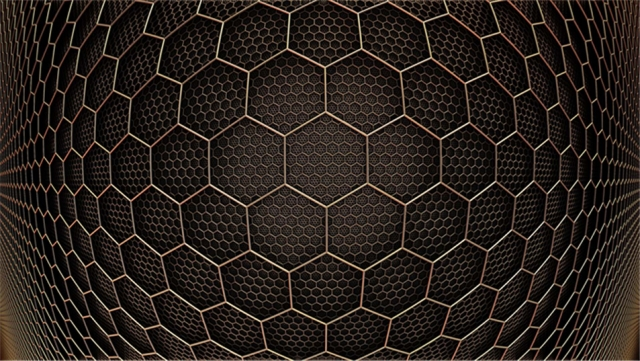# Hexagons – 720º

A regular hexagon is a six-sided regular polygon.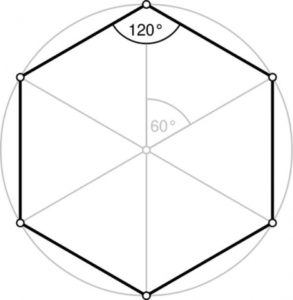Each angle = 120º

Sum of angles = 720º

720 hertz = F#.  This is two octaves up from the triangle (180 Hz) and 1 octave up from the square/circle (360 Hz).

# Recall the importance of the number 720:

• 72 is the preeminent Precessional number.
• 72 is number of years required for the equinoctial sun to complete a precessional shift of one degree along the ecliptic. This means 72 years = 1 “day” of Precession of the Equinoxes.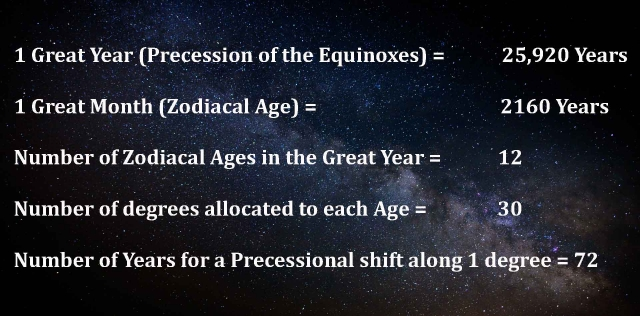• 72 = The exterior angles of a regular pentagon.
• 72 = # of edges in a truncated cuboctahedron & disdyakis

• 720 = sum of the angles of a hexagon
• 720 = sum of angles of a tetrahedron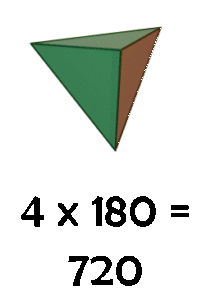• 720 x 2 = 1440 = sum of angles in a regular decagon, octahedron, and star
• 720 x 5 = 3600 = sum of angles in an icosahedron, cuboctahedron, truncated
• 720 x 6 = 4320 = sum of angles in a tetrakis hexahedron and triakis
• 720 x 9 = 6480 = sum of the angles of a dodecahedron; sum of angles of triangle thru decagon.
• 720 x 11 = 7920 = Diameter of the Earth in miles.
• 7920 = sum of degrees in the rhombicuboctahedron, snub cube, truncated octahedron, and truncated

• 720 x 12 = 8640
• 8640 = sum of degrees in the trapezoidal icositetrahedron, and disdyakis dodecahedron.
• 8640 x 10 = 86400 = number of seconds in a day.
• 8640 x 100 = 864000 = diameter of the Sun in miles.

• 720 x 14 = 10080 = sum of angles in an icosidodecahedron
• 720 x 15 = 10800 = sum of angles in a pentakis dodecahedron, triakis icosahedron, and rhombic triacontahedron.
• 720 x 18 = 12960 = sum of angles in a pentagonal
• 720 x 20 = 14400 = sum of all five Platonic
• 720 x 23 = 16560 = sum of angles in a truncated
• 720 x 29 = 20880 = sum of the angles of 4 individual Archimedian solids: (rhombicosidodecahedron, snub dodecahedron, truncated icosahedron, truncated dodecahedron)
• 720 x 45 = 32400 = sum of angles in a pentagonal hexecontahedron.
• 720 x 59 = 42480 = sum of angles in a truncated icosidodecahedron
• 720 x 210 = 151200 = sum of all 13 Catalan solids
• 720 x 230 = 165600 = sum of all 13 Archimedian solids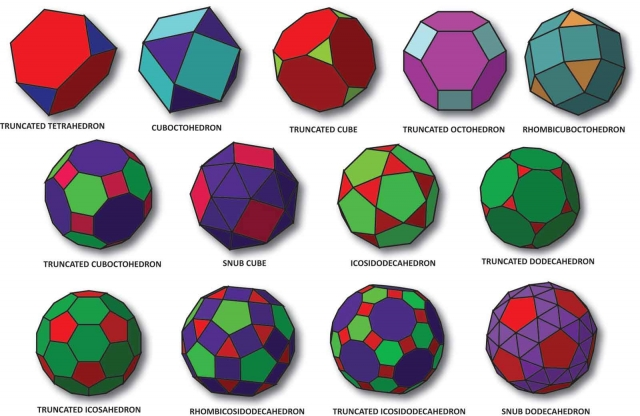13 Archimedean solids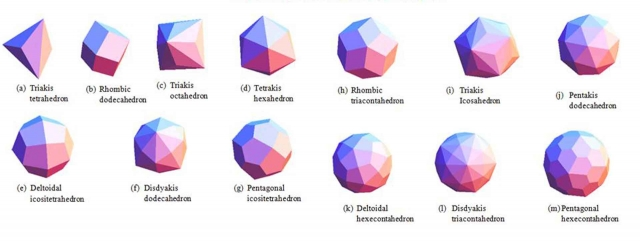13 Catalan solids

# The Hexad/Hexagon Represent the Following Qualities:

Note that these qualities exist simultaneously, never separate.

## Structure-Function-Order

The structure of any form or event determines how it can function.  Its functioning takes place in an orderly sequence.  This order of unfolding determines what the structure must be.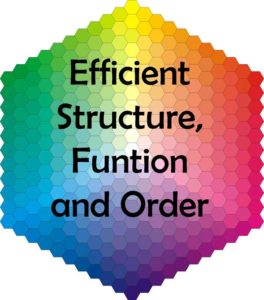## Space-Power-Time

Everything has a spatial structure, inherent power & duration in time.

“Every hexagon is a statement about the relationship of a circle’s circumference to its radius.  A hexagon is the circle’s radius externalized, its circumference internalized.  In other words, each one of a hexagon’s sides is equal to its own radius and to the radius of the circle circumscribing it.”1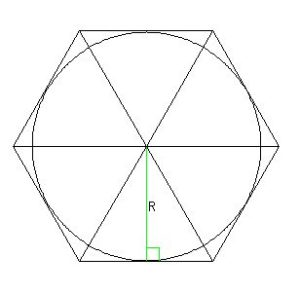Hence, the hexagon is the simplest perfect polygon to draw with only compass and straight-edge.

All you need to do is to draw a circle, keep your compass set at the circle’s radius, and use that setting to mark off 6 equally-spaced points around the circle.

Connect the points and you have your hexagon.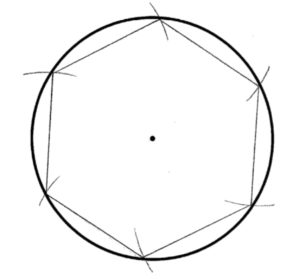Reference Construction Lesson #66: Hexagons & Hexagrams.

In the previous article we saw how the hexagon shows up in chemistry, nature and astronomy.  Now we will look at the use of hexagons in design.

# Hexagons in Design

Hexagons are used in situations requiring strength, stability & straight lines, using the fewest materials possible.

Some of these include:

Nuts & boltsWeaving patterns & woven basket centersFaucet handlesHexagonal structure of ball caps, beach balls, parachutes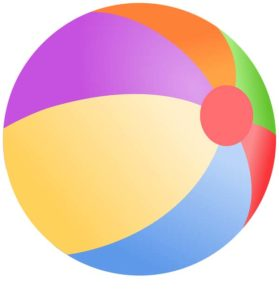Spokes of a bicycle – multiple of 6 (usually 36) provides the least weight and wind resistance with the greatest strengthDiaphragm of a camera lens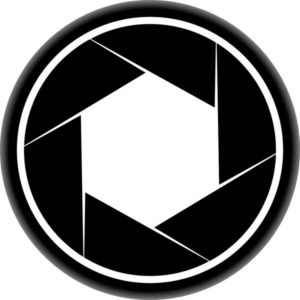Computer circuit chipChicken wireFishing netsCentral marketplaces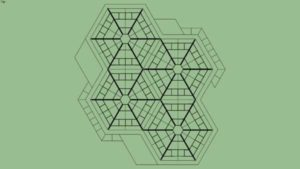“Studies find that older cities around the world seem purposefully arranged in a hexagonal lattice, a pattern that economizes market centers, decreases competition, minimizes transportation distances, and maximizes administrative control of outlying areas.”2

# Parts of a Hexagon

The length of the hexagon’s side is equal to its radius.

Its diagonal is twice the radius.The distance between opposite sides (base to roof, for example) = √3.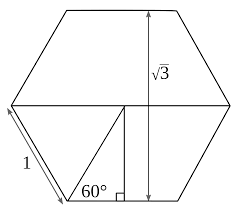Remember, when the radius of the circle = 1, the height of the Vesica Piscis is √3.  The height of a hexagon is also √3.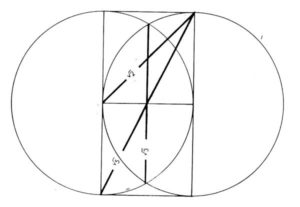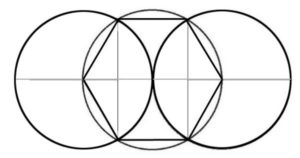We get sides 1, 2, √3 – related to the circle, line and equilateral triangle.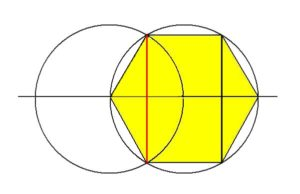We can see how the hexagon geometrically contains the principles of the Monad, Dyad, & Triad.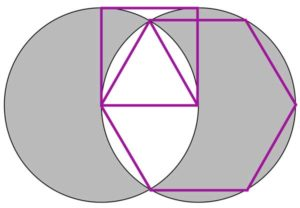# Area of a Hexagon

A = (3√3 a2)/2                             where a = side length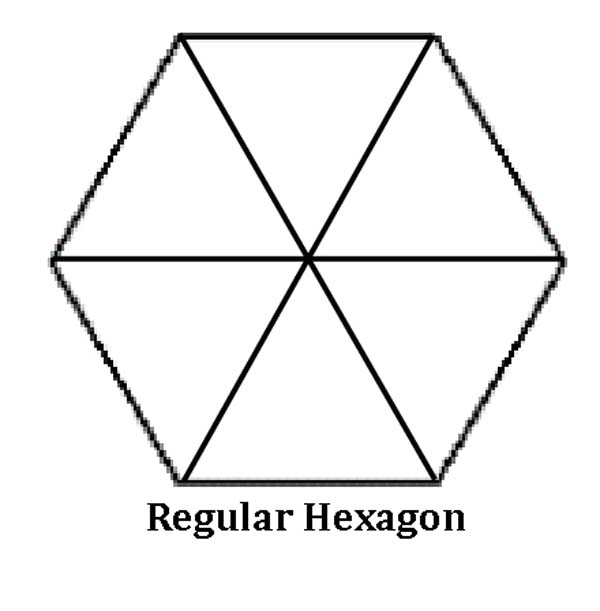The hexagon and cube are intimately related. The 2D version of a cube is a hexagon. Also, cross-sections of a cube produce hexagons when the cube is intersected by a plane perpendicular to its diagonal and is cut in half.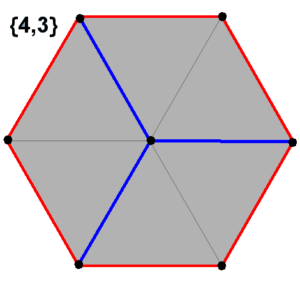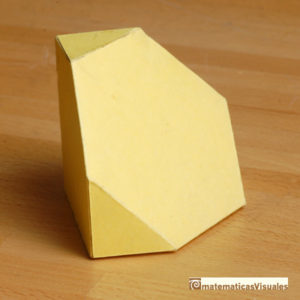The cross section of a rhombic dodecahedron is also a hexagon:There are four hexagons to be found within the cuboctahedron – the dual of the rhombic dodecahedron.  These are seen below in four different colors: red, yellow, purple and blue.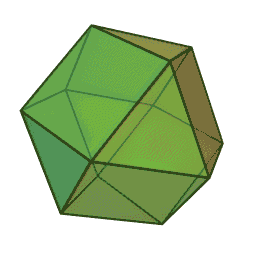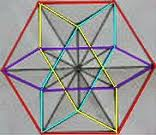# The Sound of Six

You can hear what a hexagon sounds like if you construct a hexagon on wood and hammer nails partly in at 3 corners (C, B, F shown below) and tie a guitar string or nylon fishing line around the nails, keeping equal tension between CB, BF and CF.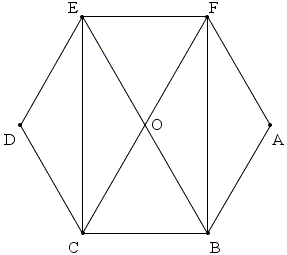The shorter the string, the higher the tone.

The three form a harmony (1, 2 √3) characteristic of the hexagon.

# Hexagrams

There is a single stellation of the hexagon: the hexagram.  The hexagram is a six-pointed star.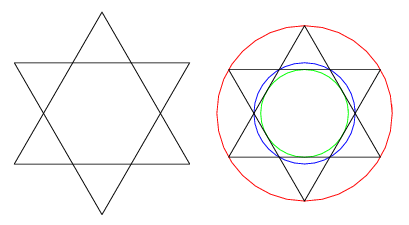The 3D version of the hexagram is the star tetrahedron.  The cross-section of a star tetrahedron is a hexagon.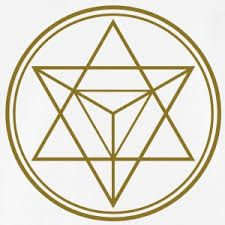It is a 2D representation of the star tetrahedron (the shape of the confined photon).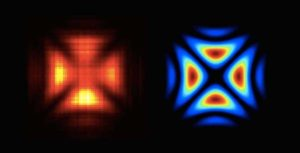Captured image of a single confined photon (on left).  It looks like a Maltese Cross.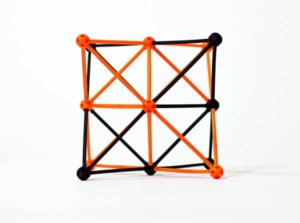The star tetrahedron seen from its ‘cubic’ angle.  The cross is clearly seen.

# The Hexagram in Religion

The hexagram shows up widely in religion and myth

Read here to find out the origins of Sat-kona [the Star of Goloka or Goloka-yantra], its transcendental significance, its historical use in Vedic and other ancient cultures, its use in Christianity and Islam, and its eventual adoption by the Jewish faith in the 17th century as a popular symbol of Judaism.

Star of David – Judaism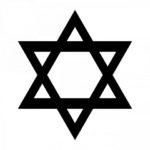Seal of Solomon (Ring of Solomon) – Muslim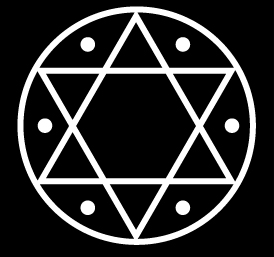Shatkona Yantra (Mark of Vishnu) – Hindu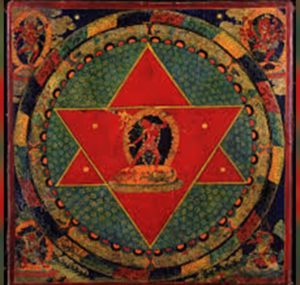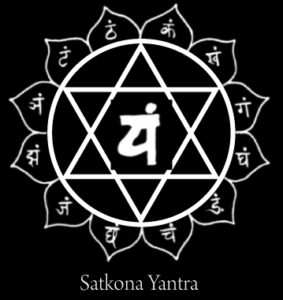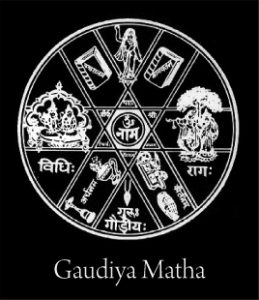Buddhism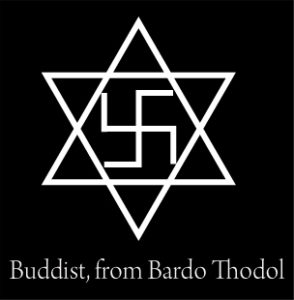Vajravarahi Yantra – Dzogchen Buddhism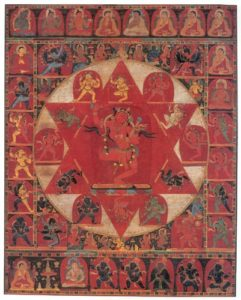Jain Yantra – Jainism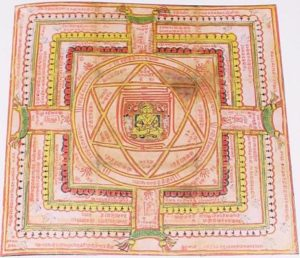Chrismon – Christian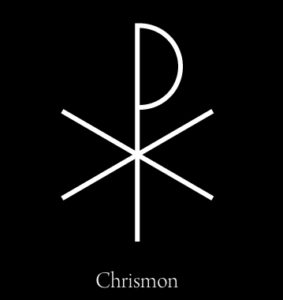Merkaba (light body) – Egyptian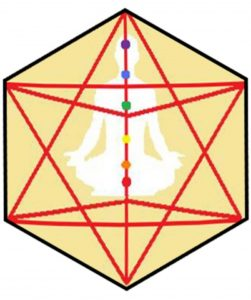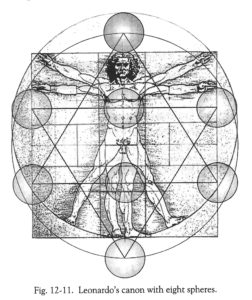Compass & Rule – Freemasonry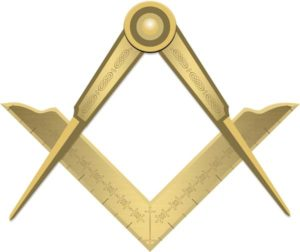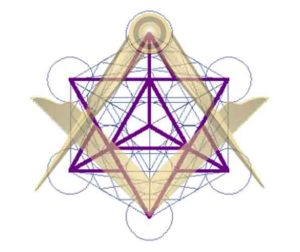Ancient Mystery Traditions – “The great seal of initiates, signifying rising above aspiration from below met by the descent of grace from above.”3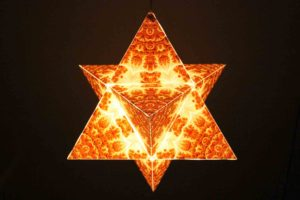Theosophy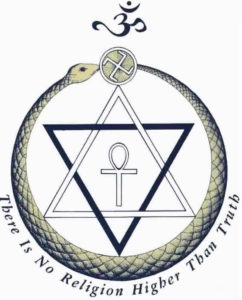# The Hexagram and the Octave

A circle constructed within the inner hexagram has a circumference exactly half of the large outer circle.This is seen in the inner green circle to the red outer circle in this diagram.

This is a visual representation of the Octave (1:2).

# The Seed of Life and the Hexagon

This shape of 6-around-1 is commonly known as the “Seed of Life”.  Keith Critchlow calls it the “Flower of Creation”.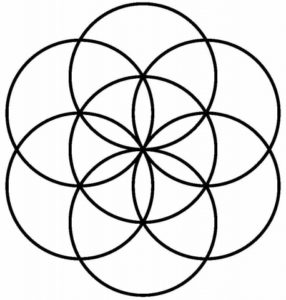There are many hexagons within hexagons that can be drawn from the intersections of these circles.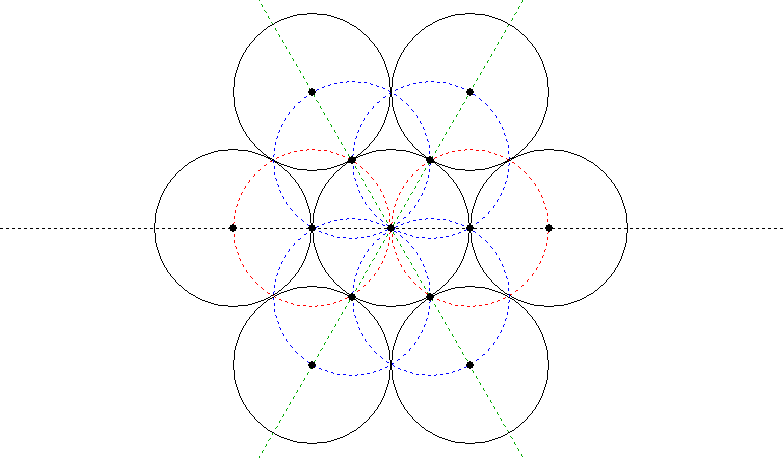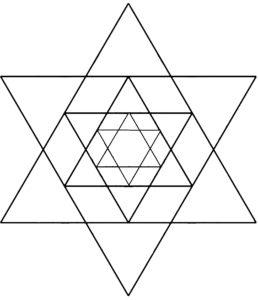# Division of a Circle into 6 Parts Using a Rhomb

A 60º rhomb (pictured below) can be used as a template to divide a circle into 6 equal parts.This is seen in the smallest Bronze Age gold breastplate found at Bush Barrow, near Stonehenge (pictured below).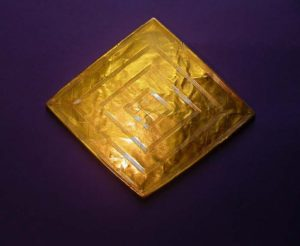Placing the point of the rhomb at the center of a circle will divide the circle into 1/6.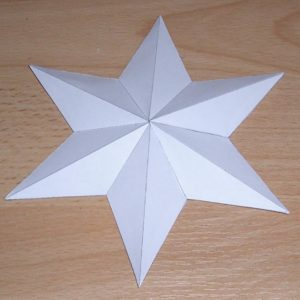This is discussed extensively in Keith Critchlow’s Time Stands Still.

# The Vesica, 5 and 6 – Union of the Hexagon and Pentagon

This geometric construction was originally published by Albrecht Durer in Course in the Art of Measurement with Compass and Ruler.

It was later republished in Charles Bouleau’s The Painters Secret Geometry & John Michell’s The City of Revelation.

This construction symbolizes the emergence of the Universe (6) and Life (5) from the cosmic womb of creation.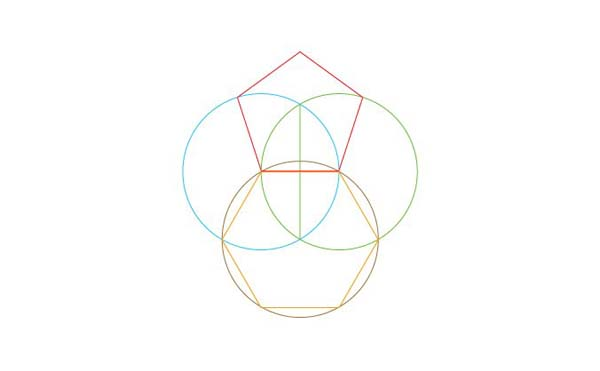“The reconciliation of six and five and many of the other objects of sacred geometry may be achieved by means of the figure known as the Vesica piscis.”4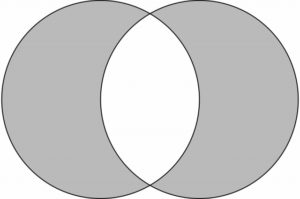Master geometer Randall Carlson remarks of it, “The reconciliation of six and five refers to the potential to develop, from the Vesica as a starting point, polygons of six and five sides, as well as many of the other polygons, with all their symbolic connotations and unique properties.”

The hexagon is easier to draw and precedes the pentagon.

This represents how the cosmos had to exist before humankind could emerge from the combination of matter and energy generated through the evolution of the universe.

The pentagon has psychic and lunar qualities.

The hexagon has rational and solar qualities.

The three angles of an equilateral triangle, six of which make up a hexagon, are each of 60 degrees, reflecting the solar number 666.

The pentagon angle is 108 degrees, 1080 being the lunar number as the radius of the Moon is 1080 miles.

According to John Michell, the pentagon and hexagon combined also symbolizes “the purpose of a true scholar, to relate his own interests to those of the world at large…As a symbol of reconciliation between different orders in nature, its presence here prepares the student of life’s mysteries for the contradictions and paradoxes he/she will encounter and provides the geometric model to contain them.”5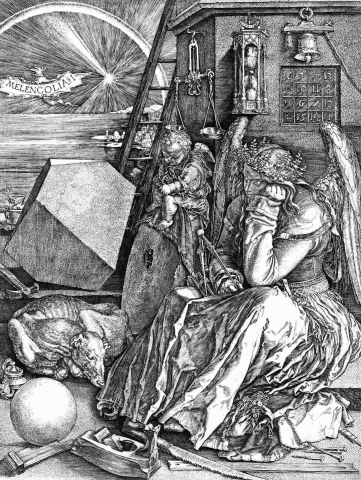Albrecht Durer’s Melancholia I

Reference Construction Lesson #9: The Vesica, Hexagon & Pentagon.

1. Schneider, Michael, A Beginner’s Guide to Constructing the Universe, Harper Perennial, 1994
2. ibid.
3. ibid.
4. Michell, John, The Dimensions of Paradise, Inner Traditions, 2nd edition, 2008
5. ibid.

Return to Free Library

Return to Number Menu